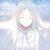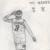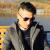## 求大神们帮忙修改正则表达式

mr桀骜不驯 发布于 2014/09/16 16:10

1、一个字母接上0到3个数字（如'A123'，'A12'，'A1'，'A'）

2、1到4个数字（如'1234'，'123'，'12'，'1'）

3、一个字母接上1到2个数字再接上一个字母（如'A12A'，'A1A'）

4、一个数字接上“-”再接上1到2个数字（如'1-12'）

5、以上格式重复0到2次，以逗号隔开（如'A123'，'A23,A234'，'A23,A23A,234'）

^((([A-Za-z]\\d{0,3})|\\d{1,4}|([A-Za-z]\\d{1,2}[A-Za-z])|(\\d-\\d{1,2}))(,(([A-Za-z]\\d{0,3})|(\\d{1,4})|([A-Za-z]\\d{1,2}[A-Za-z])|(\\d-\\d{1,2}))){0,2})\$

0
0([A-Za-z]\d{0,3})(\d{1,4})([A-Za-z]\d{1,2}[A-Za-z]\d-\d{1,2})  逗号分隔无所谓，取匹配数量就可以了0